Open in App
Not now

# Density Plots Using Lattice Package in R

• Last Updated : 30 Dec, 2022

The density plots are mainly used to visualize the distribution of continuous numeric variables. The densityplot() uses kernel density probability estimate to calculate the density probability of numeric variables. In R Programming, the density plot can be plotted using the densityplot() function which is present in the lattice package.

Syntax: densityplot(x,data, type, xlab, main)

where,

• x – the column(s) where the density needs to be visualized
• data – data frame is specified here
• xlab – used to add an x-axis label to the plot
• main – used to add a title to the plot

In this article, we will be using the iris dataset. Firstly, we will plot the Simple Density Plot. Before that, install the lattice package using the below code.

## R

 `# Install the required package``install.packages``(``"lattice"``)``# Load the installed package``library``(lattice)`` ` `# Load the default dataset(iris) into current working environment ``data``(iris)`` ` `# Plotting the Simple density plot using densityplot()``lattice::``densityplot``(~Sepal.Length, data=iris,``                     ``xlab=``"Sepal Length"``,``                     ``main=``"Simple Density Plot of iris Dataset"``)`

Output: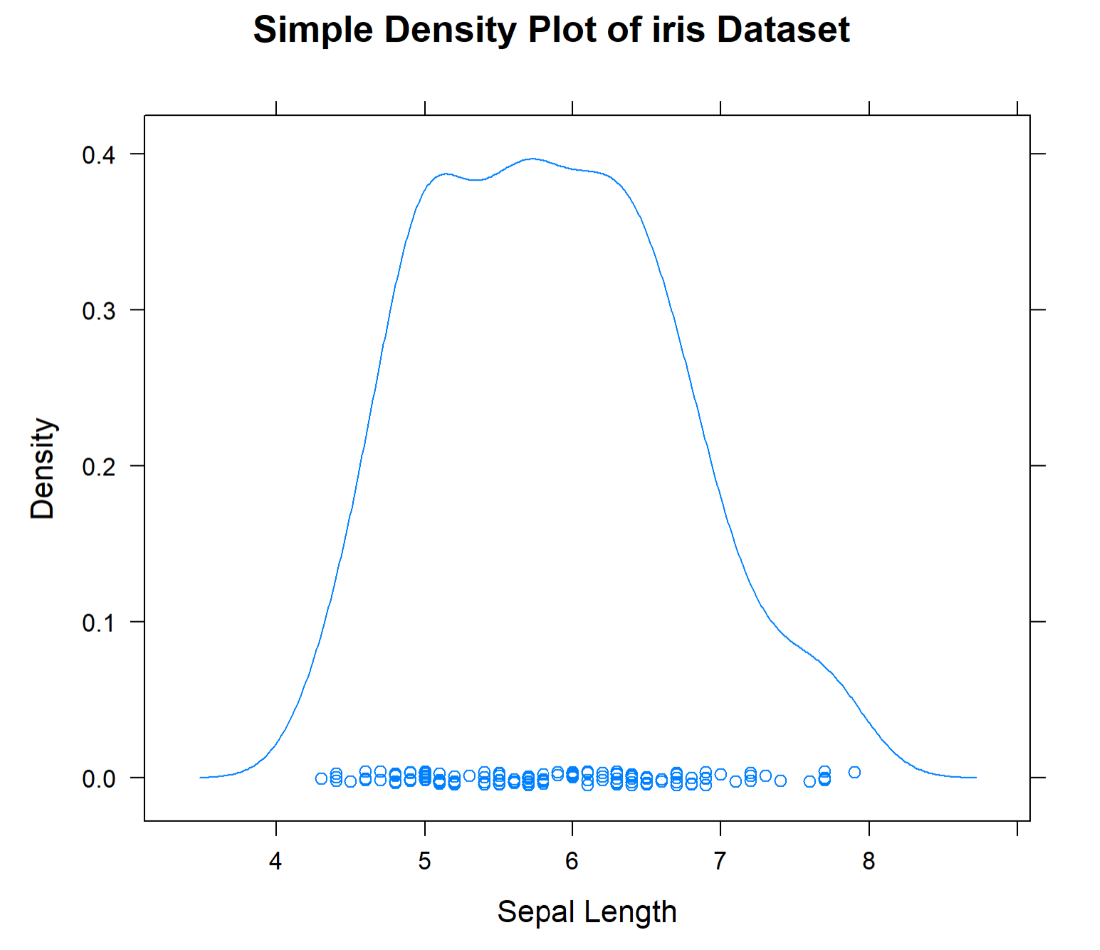We can also specify the particular points using parameters from and to. The output will be same as above but only between the points we have specified.

## R

 `# Using from and to``lattice::``densityplot``(~Sepal.Length, data=iris,``                     ``xlab=``"Sepal Length"``, main=``"Iris Dataset"``,``                     ``from=4, to=6)`

Output :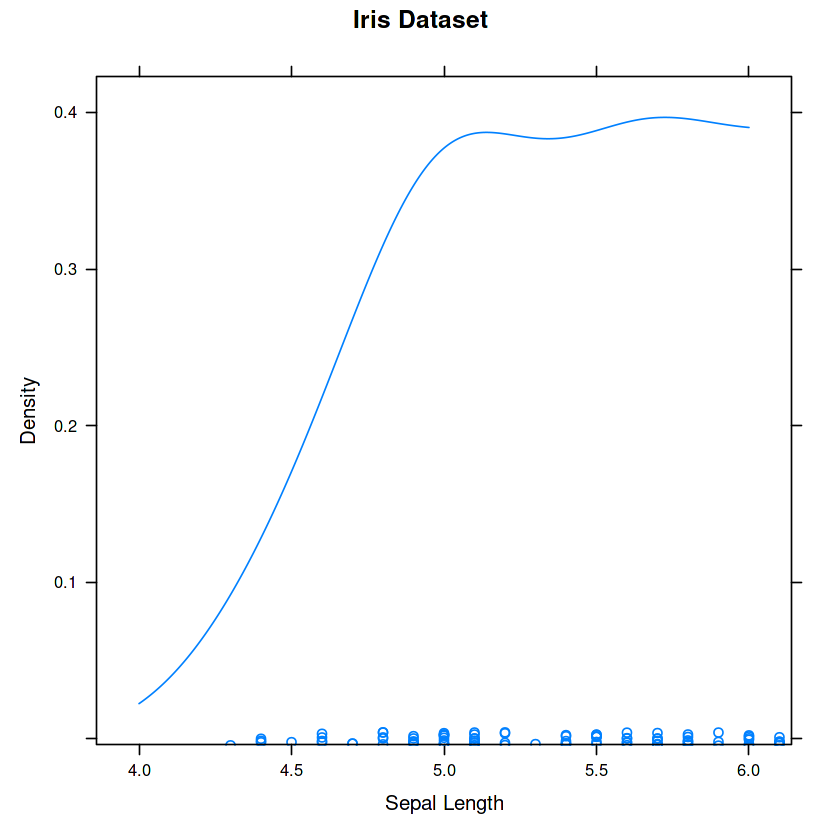To plot the partitioned density plot as per the Species Category, follow the code given below.

## R

 `# Plotting the Partitioned density plot using densityplot()``lattice::``densityplot``(~Sepal.Length|Species, ``                     ``data=iris, layout=``c``(1,3),``                     ``xlab=``"Sepal Length"``,``                     ``main=``"Partitioned Density Plot of iris Dataset"``)`

Output: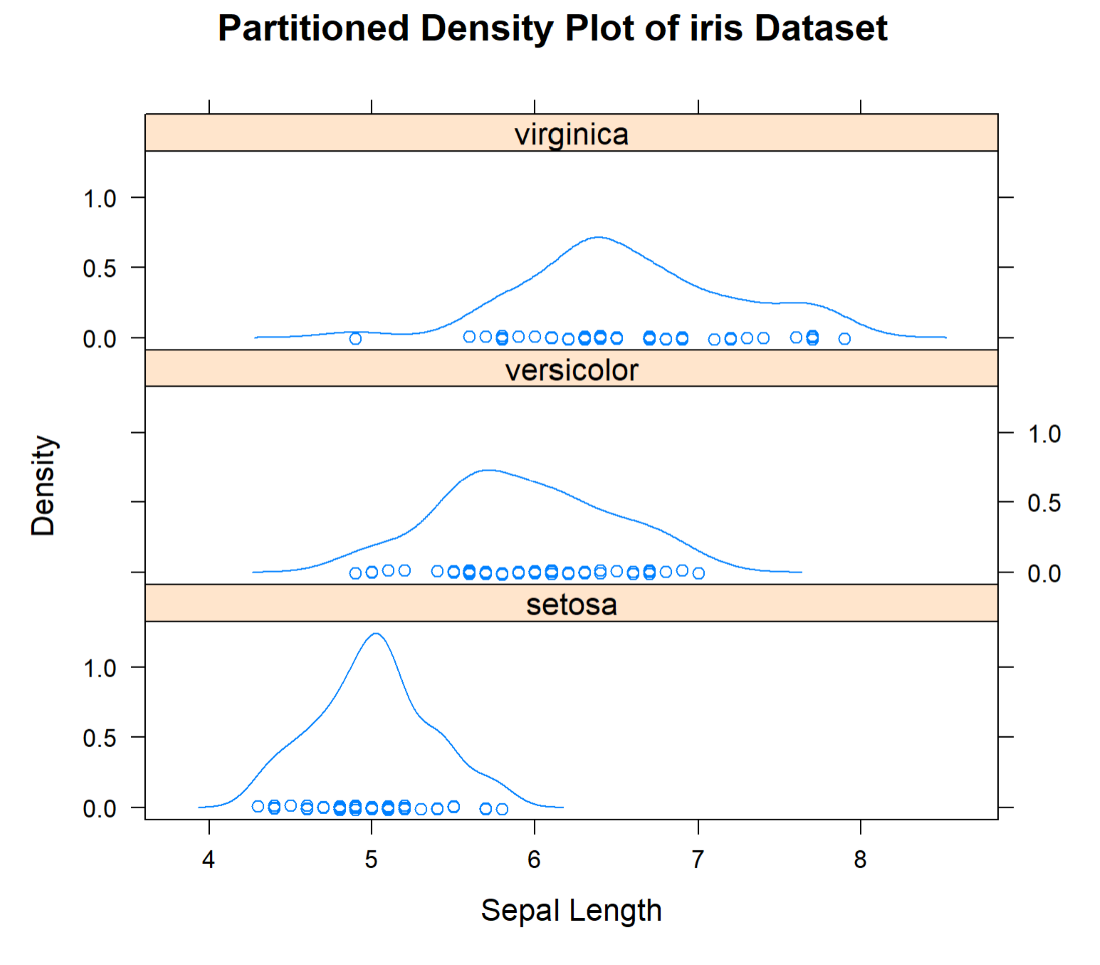We can also plot the Count Based Density Plot by specifying the type parameter as count.

## R

 `# Plotting the count based density plot using densityplot()``lattice::``densityplot``(~Sepal.Length|Species, ``                     ``data=iris, layout=``c``(1,3), ``                     ``type=``"count"``, xlab=``" Sepal Length"``,``                     ``main=``"Count Based Density Plot of iris Dataset"``)`

Output: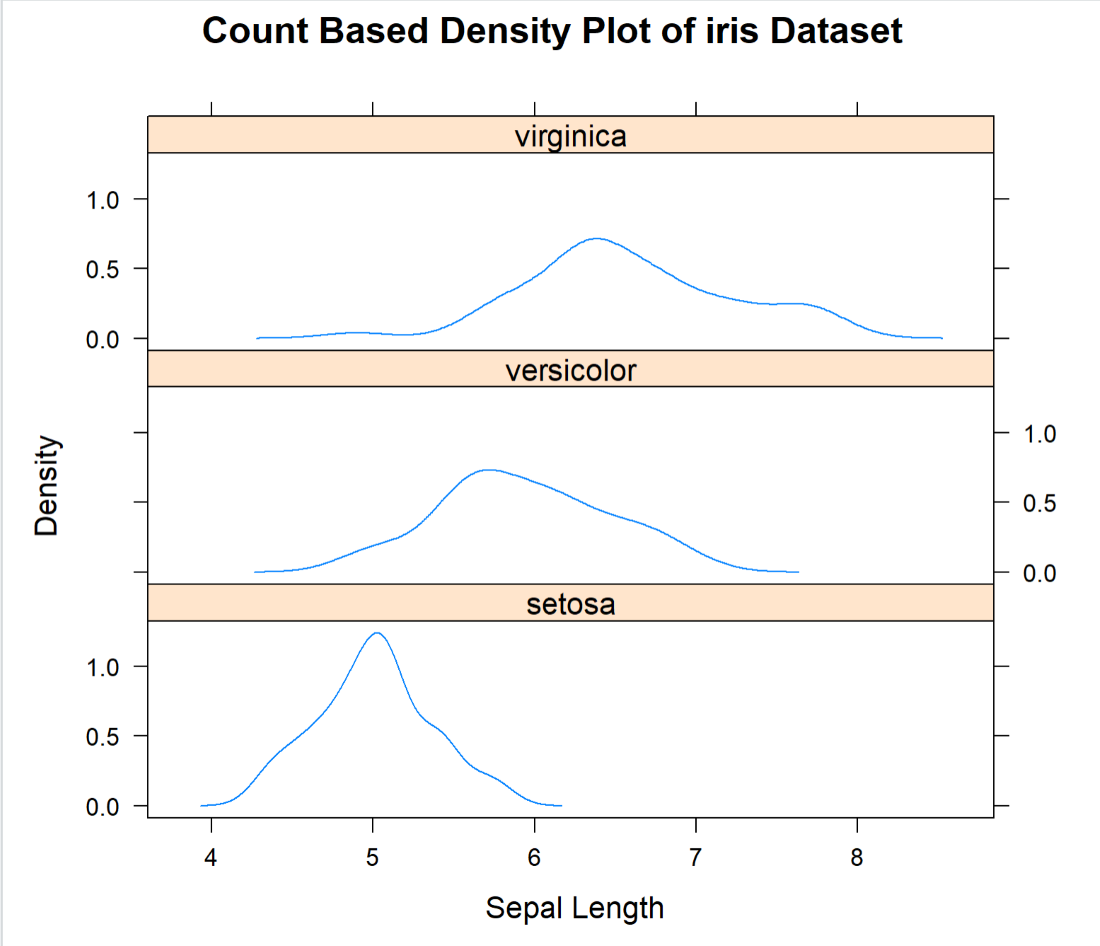Let’s see how to plot Customized Density Plot for iris dataset.

## R

 `# Plotting the Customized density plot using densityplot()``lattice::``densityplot``(~Sepal.Length|Species, data=iris,``                     ``layout=``c``(1,3), xlab=``"Sepal Length"``,``                     ``main=``"Customized Density Plot of iris Dataset"``,``                     ``col=``c``(``"red"``,``"blue"``,``"green"``))`

Output: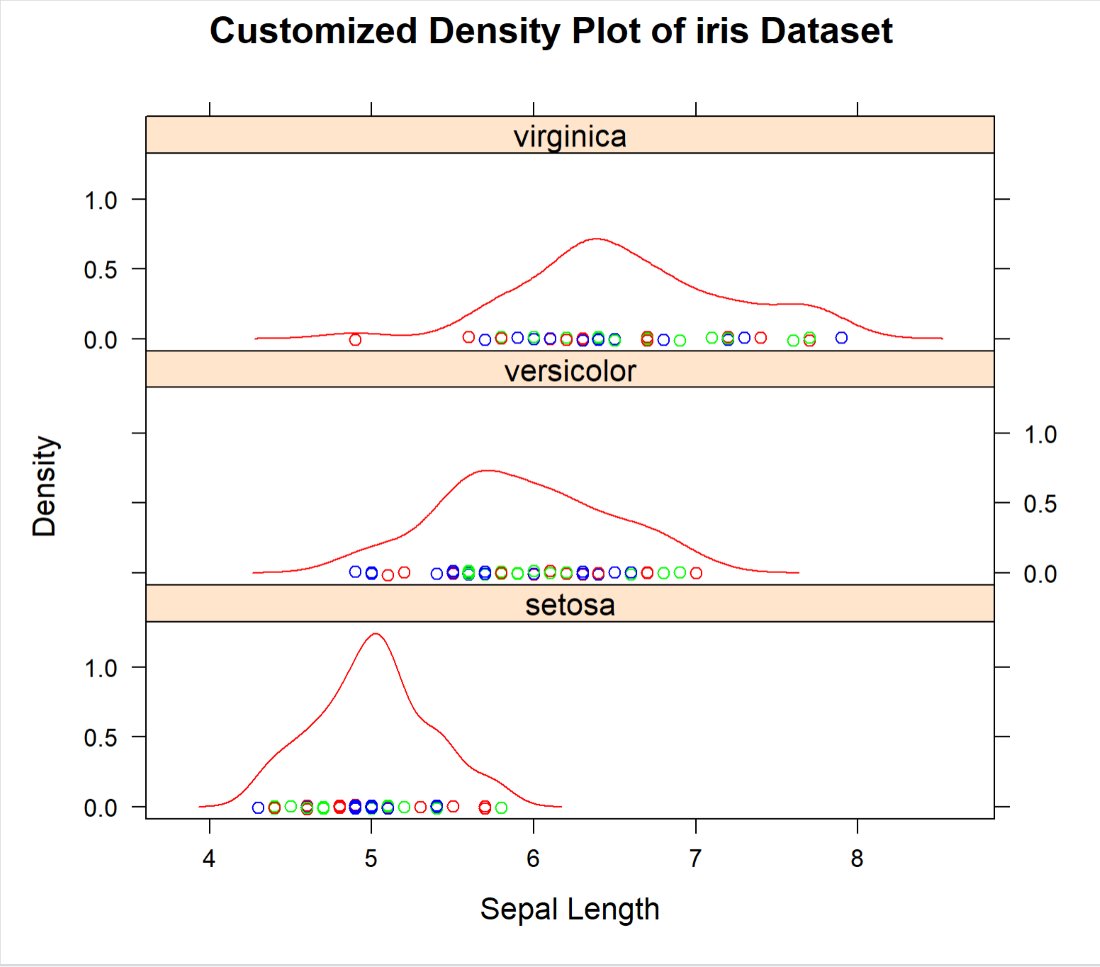My Personal Notes arrow_drop_up